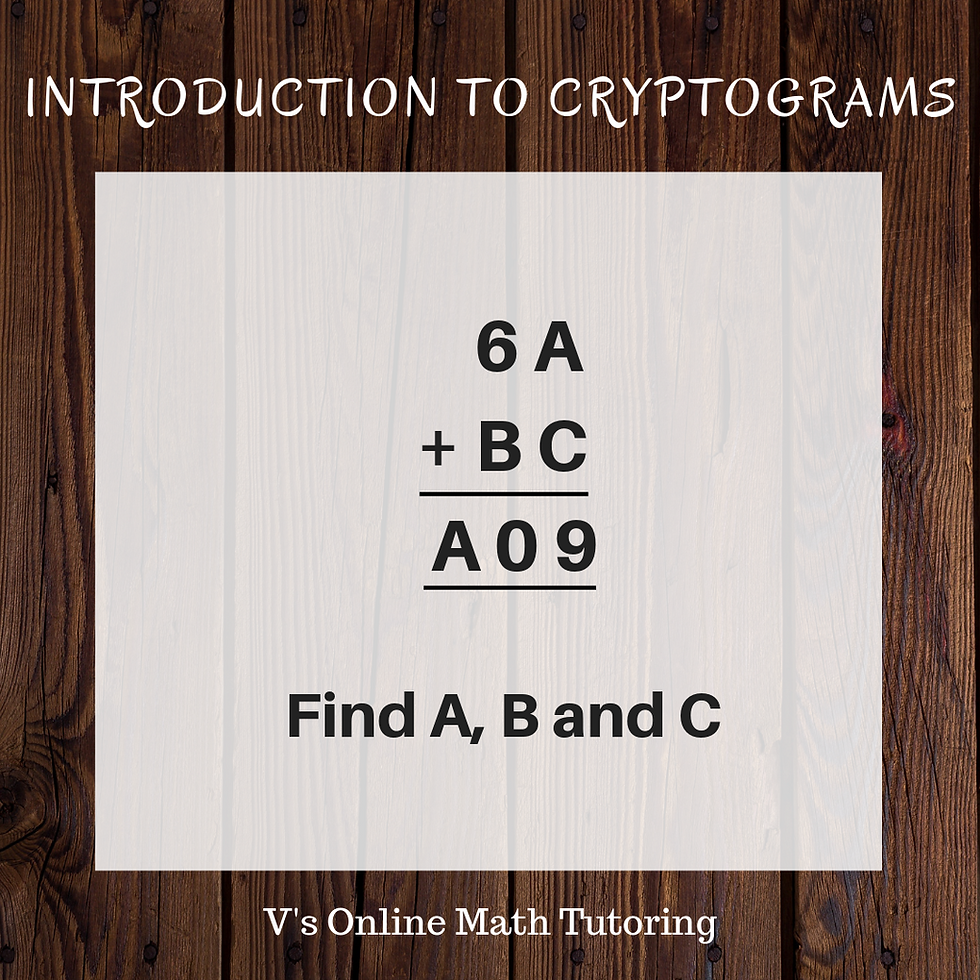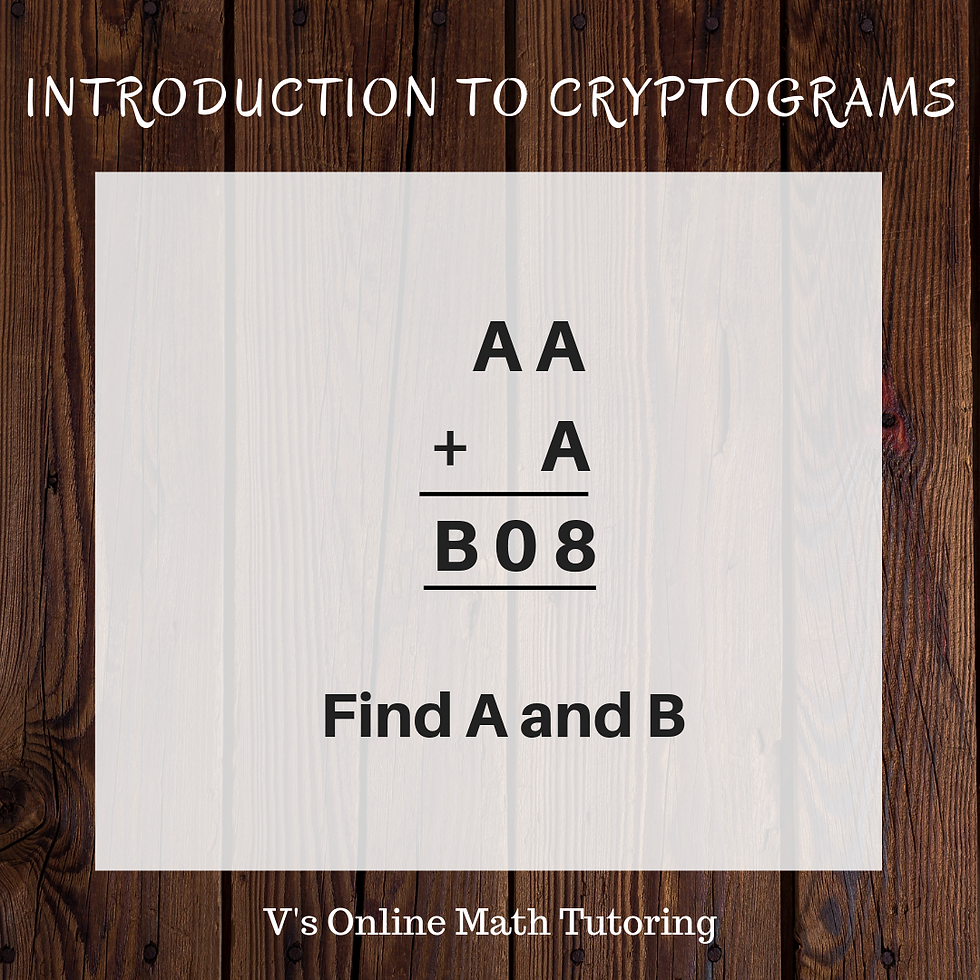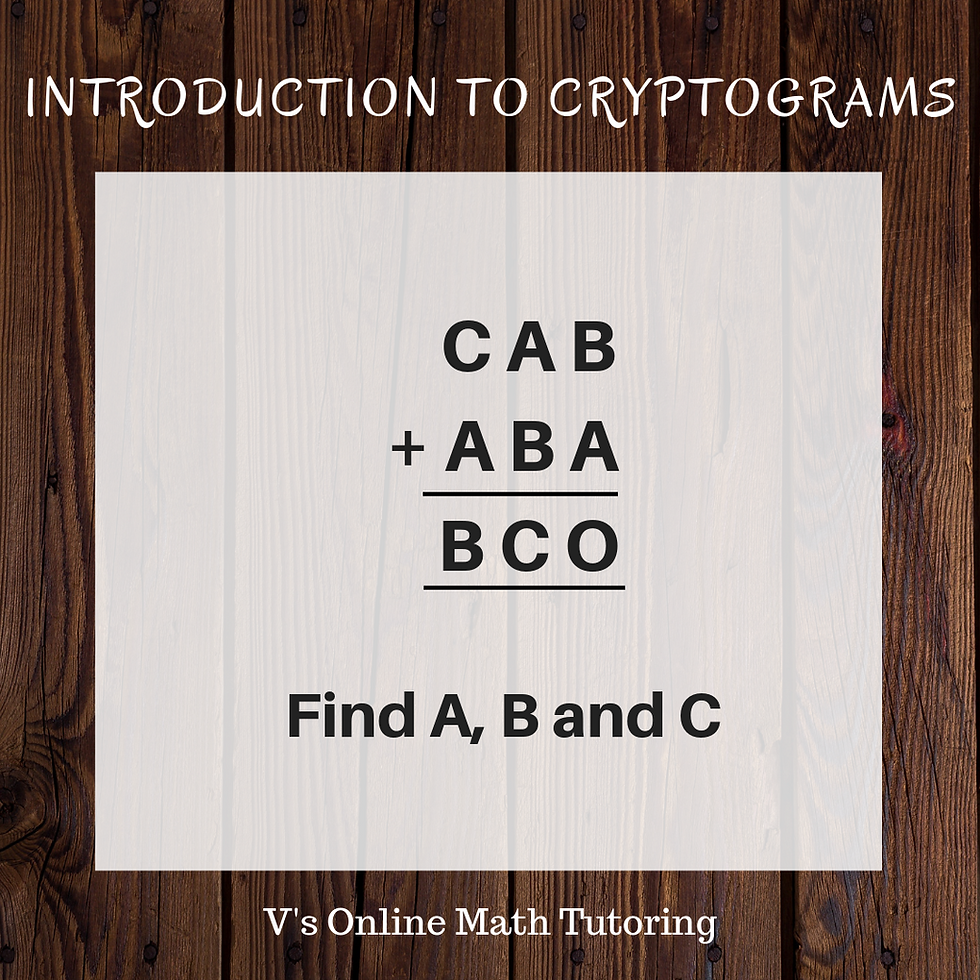top of pageSearch

# Math Enrichment Activites - Cryptogram/Cryptarithm Math Puzzles and How to solve them

Updated: May 18, 2021

A Cryptogram/ Cryptarithm is a puzzle where letters and symbols are used in place of numbers. Our goal is to figure out which number each letter and symbol corresponds to. These are very popular in mathematical competitions especially in MOEMS (Math Olympiads for Elementary and Middle Schools) and CML (Continental Math League). In fact CML competition gives cryptograms to kids as young as second grade. Cryptarithm puzzles are also engrossing and interesting to solve. They give our brain a good workout.Cryptogram puzzles for Elementary and Middle School Kids

I also do these puzzles with my 5-year old kid and my students (as a part of my Math Enrichment program) and they enjoy it. The puzzle given above has been one of my more popular puzzles because I posted it around Halloween with the word BOO :) and it's easy to solve.

So now let's look at various cryptogram puzzles and how to solve them

Let us start with the most basic one. Like I said each letter stands for a single digit number and different letters represent different numbers.

Puzzle 1: For kids in grades 1-2Cryptarithm Puzzle 1

So here we have

5+B=; 5+1=6 hence B=1

A+3=7; 4+3=7 hence A=4

45+31=76 and that verifies our answer

So our solution is A=4; B=1

Puzzle 2: For kids in grades 1-2

Here we introduce the idea of carryoversCryptarithm Puzzle 2

It's given that 8+B=7; but 8 plus B (a single digit whole number) cannot equal 7 and hence it must 8+B should be equal to 17; and the 1 would be carried over.

So, 8+B=17; 8+9=17; hence B=9

Now we have a carryover of 1 in the ten's place

So, 1+A+2=6; A+3=6; 3+3=6; hence A=3

We know that 38+29=67 and hence out solution is correct

So we have A=3 and B=9

Puzzle 3: For kids in grades 2-3Cryptarithm Puzzle 3

A+C=9; It could be any possible answer ranging from 0+9=9 to 4+5=9. So how do we narrow it? There's an A in the hundred's place in the sum. A's value has to be the same in both places. So let's see if we can figure out the A in the hundred's place

When 2 2-digit numbers are added the greatest carry over you can have in the hundred's place is 1.

eg. let's take the work case and add 99+99 = 198.

So A has to be 1.

The other way to look at it is 6+B=0 can have a greatest carryover of 1. When 2 single digit numbers are added (highest being 9+9=18) you can only have a carryover of 1. Hence A=1

Now that we know A=1; rewrite your problem as 61+BC=109

Now we have 1+C=9; 1+8=9; hence C=8

We also have 6+B=10; 6+4=10; hence B=4

61+48=109, it works and our solution is correct

Solution: A=1; B=4; C=8

Puzzle 4: For kids in grades 3-5Cryptarithm Puzzle 4

Pretty similar to Puzzle 3. Figuring out B is easy because B is in the hundred's digit and it can only be 1.

Pitfall to watch out for: It is tempting to say that A=4 because 4+4=8. But then if A=4, the tens column would not be correct. 4+0 cannot be 0. So there needs to be a carryover. Hence A+A should be equal to 18. Hence A=9

99+9=108; So our solution is A=9; B=1

Puzzle 5: For kids in grades 3-5Cryptarithm Puzzle 5

A+A+A = 4; which means 3A=4; since 3*A cannot be equal to 4, it has to be equal to 14 or 24. (When 3 single digit numbers are added the greatest carry over is 2; eg. 9+9+9=27)

3*8=24; Hence A=8

We have a carryover of 2; so 2+2+3=B; and B=7

28+38+8=74 and it works

So out solution is A=8 and B=7

Now its your turn to try. Try this problems and write the answers in the comments section belowCryptarithm Puzzle 6

Like these Cryptograms? You can find a lot more of these if you follow my Facebook or Pinterest page.

https://www.pinterest.com/vasudhauddavan/interesting-math-problems/P.S. I just started Cryptograms with my 5-year-old son yesterday and this will give you an idea of how to start with your own child.

I made up all these problems for him. I always hand write the problems one-by-one for him as young kids tend to get distracted when there see too many problems to solve.

You can also see how I started from extremely easy problem of A+5=8 and moved on to 2-digit addition with carryovers. We also later did 2-digit addition with carryovers in tens and hundreds place.

This is also a good way to practice addition and subtraction. Kids love the variety.

I am Vasudha, an Online Math Tutor. I do Math Enrichment classes for Elementary and Middle School students. I will also be running Online Math Enrichment Programs during the summer.

Registrations are now open and click here to find out more... Summer Fun with Math Enrichment Activities and Puzzles

Did you enjoy these puzzles? Have a friend or a family member who'll benefit from these as well? Please do share the blog :)

## Related Posts:

Math Competition Problems - Problem of the Week 8

#ElementarySchoolEnrichment